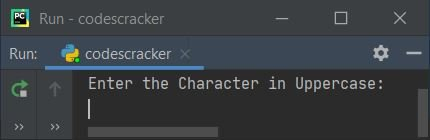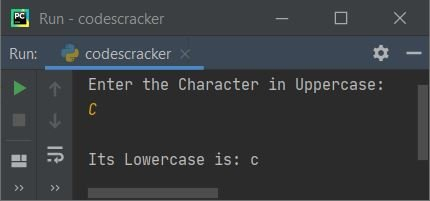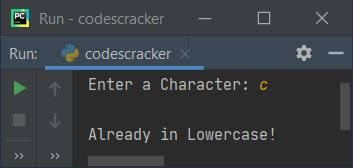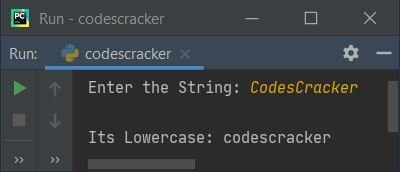# Python Program to Convert Uppercase to Lowercase

In this article, I've created some programs in Python, to convert a character or string entered by user from uppercase to lowercase. Here are the list of programs:

• Uppercase to Lowercase Character using ASCII Value
• Uppercase to Lowercase String using ASCII Values
• Uppercase to Lowercase using lower()
• Using user-defined Function
• Using Class and Object

Note - ASCII value of a is 97, b is 98, and so on upto ASCII value of z is 122. Whereas ASCII value of A is 65, B is 66, and so on upto ASCII value of Z is 90.

## Uppercase to Lowercase Character using ASCII Value

To convert uppercase character to lowercase in Python, you have to ask from user to enter a character, then convert that character to its equivalent lowercase using ASCII value as shown in the program given below:

```print("Enter the Character in Uppercase: ")
ch = input()

chlow = ord(ch)
chlow = chlow + 32
chlow = chr(chlow)
print("\nIts Lowercase is:", chlow)```

Here is its sample run:Now supply the input say C as character, press `ENTER` key to convert and prints its equivalent character in lowercase as shown in the snapshot given below:Note - The ord() function returns ASCII value of character passed as its argument. Whereas chr() function returns corresponding character of ASCII value passed as its argument.

For example, if user enters C as character input, then after executing the following statement:

`chlow = ord(ch)`

the ASCII value of ch gets initialized to chlow. That is, ASCII value of C (67) gets initialized to chlow. So chlow=67. Now using the following statement:

`chlow = chlow + 32`

chlow + 32 or 67 + 32 or 99 gets initialized to chlow. So chlow=99 now. And 97 is the ASCII value of c. Therefore after executing the statement given below:

`chlow = chr(chlow)`

The corresponding character to ASCII value 99 gets initialized to chlow. So chlow = c. In this way, the uppercase character gets converted into lowercase.

#### Modified Version of Previous Program

This program handles invalid input too. The end= used in this program, to skip inserting an automatic newline.

```print("Enter a Character: ", end="")
ch = input()

chlen = len(ch)
if chlen==1:
if ch>='A' and ch<='Z':
chlow = ord(ch)
chlow = chlow + 32
chlow = chr(chlow)
print("\nIts Lowercase is: ", chlow)
elif ch>='a' and ch<='z':
else:
print("\nInvalid Input!")
else:
print("\nInvalid Input!")```

Here is its sample run with user input c:## Uppercase to Lowercase String using ASCII Values

This program works similar to previous program. In this program, each and every character of given string gets checked and converted to lowercase, one by one.

```print("Enter the String: ", end="")
text = input()

textlen = len(text)
for i in range(textlen):
if text[i]>='A' and text[i]<='Z':
ch = text[i]
ch = ord(ch)
ch = ch + 32
ch = chr(ch)
text = text[:i] + ch + text[i+1:]

print("\nIts Lowercase:", text)```

Here is its sample run with user input, CodesCracker:The following statement:

`text = text[:i] + ch + text[i+1:]`

states that the new string gets initialized as new value of text, after converting the current index's character to lowercase. In this statement, text[:i] refers to all characters from 0th to (i-1)th index. And text[i+1:] refers to all characters from (i+1)th to (len-1)th index. Here len is the length of string.

For example, if text = "CODEScracker", i=5, and ch="C" therefore the following statement:

`text = text[:i] + ch + text[i+1:]`

gets evaluated like this, after putting the values:

`text = "CODES" + "C" + "racker"`

that is, "CODESCracker" gets initialized to text as its new value.

Note - While slicing sub-string from string using indexing, that is [:]. The index number before colon refers as included index, whereas the index number after colon refers as excluded index.

## Uppercase to Lowercase using lower()

This program converts uppercase to lowercase string or character using predefined function of Python named lower():

```print("Enter the String: ", end="")
text = input()

text = text.lower()
print("\nIts Lowercase:", text)```

## Uppercase to Lowercase using Function

This program is created, using a user-defined function named UpToLow(). This function receives a value as its argument and returns its lowercase using lower() method:

```def UpToLow(u):
return u.lower()

print("Enter the String: ", end="")
text = input()

text = UpToLow(text)
print("\nIts Lowercase:", text)```

## Uppercase to Lowercase using Class

This program is similar to previous program. The only difference is, this is created using class, an object-oriented feature of Python.

```class CodesCracker:
def UpToLow(self, u):
return u.lower()

print("Enter the String: ", end="")
text = input()

ob = CodesCracker()
text = ob.UpToLow(text)
print("\nIts Lowercase:", text)```

To access any member function of a class, an object must be created. Therefore I've created an object named ob of class CodesCracker. Now using this object, I've accessed the member function named UpToLow() using dot (.) operator.

#### Same Program in Other Languages

Python Online Test

« Previous Program Next Program »